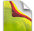### Feel The Power# PHP If else Statements

One of the single most important statements in every programming language is the "if" statement. Being able to set up conditional blocks of code is a fundamental principal of writing software. If you have already used another programming language, chances are that you can use the PHP if statement straight away, since it looks pretty much the same as in other programming languages. If not, here are some examples to get you started.

``````<?php
\$number = 42;
if(\$number == 42)
echo "The number is 42!";
?>``````

It's very simple. We declare a variable called number and assign the value 42 to it. In the next line, we use the if statement to check if the number is what we expect. The next line of code will only be executed if the condition evaluates to true. If you change the number in the first line to something else, you will see that the echo line is not executed.

As you can see, we are using two equal signs after each other - why is that? In PHP, and in many other programming languages, a single equal sign is an assignment operator. It's used to assign a value to e.g. a variable. Two equal signs are a comparison operator, that is, it's used to compare two values. So, in this case, we use two equal signs to compare the two items: \$number and 42. Had we only used one equal sign, 42 would be assigned to \$number, and no comparison would have been made.

PHP actually takes it one step further, introducing the triple equal sign. The difference is if you use two equal signs, PHP will only look to see if the values are identical. If you use three of them, PHP will compare both value and type and only if both of them match, the expression is true. Here is an example to show you the difference:

``````<?php
\$number = 42;
if(\$number === "42")
echo "The number is 42!";
?>``````

All we did was compare the variable against a string containing "42" instead of the number 42. But now that we use three equal signs, the types are no longer the same, since one is an integer and the other is a text string. I'm sure that seems a bit complicated right now, but just try to remember it for now - in the beginning, the double equal sign is pretty much all you need.

## The else statement

If one of the ways is not good enough, why not go the other way? The else statement is the if statements best friend - it's the alternative to a failed if statement. In code, it can look a bit like this:

``````<?php
\$animal = "Cat";
if(\$animal == "Dog")
echo "It's a dog!";
else
echo "I'm sure it's some sort of animal, but not a dog!"
?>``````

Whenever the if statement doesn't evaluate to true, the else statement(s) are executed, if there are any. As you saw in the first example, the else part is not required if you don't need it. In this case, we look for a certain animal, the dog, and prints a status message depending on whether or not we found it. Try changing the \$animal variable between "Cat" and "Dog" to see the difference.

In this example, each conditional block only consists of one line of code. That's why we can leave out the curly brackets. In a case where more than one line of code should be dependent of our if or else statement, they should be surrounded by curly brackets, like this:

``````<?php
\$animal = "Cat";
if(\$animal == "Dog")
echo "It's a dog!";
else
{
echo "I'm sure it's some sort of animal, but not a dog!";
echo "This line is still a part of the else statement.";
}
?>``````

## Different operators

In the previous examples, we only used the equal operator to compare values. However, there are many more operators. Each of them can be used instead of the equal operator, e.g. like this:

``````<?php
\$number = 42;

if(\$number != 30)
echo "Hello World!";
?>``````

!= means "not equal to", the opposite of == (equal to)

if(\$number > 30)

Is \$number bigger than 30?

if(\$number < 30)

Is \$number smaller than 30?

if(\$number >= 30)

Is \$number bigger than or equal to 30?

if(\$number <= 30)

Is \$number smaller than or equal to 30?

#

#

#

#

### PHP Conditions Rule

TOP TUTORIALS
WHAT IS NEW IN KERIXA?
RECENT TUTORIALS
CATEGORIES